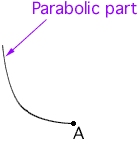A fairground's most popular attraction is a roller coaster ride known as the Terror Run. One stretch of track is called the Missile Path and is in the form of a parabolic curve. B is 180m horizontally from A and the highest point of the curve is 100m above A and B.

A.. The owner works out a quadratic expression to describe the Missile Path. What is the expression He found?

B.. A safety Engineer examined the structure and observed that points A and B were likely to be damaged due to the steepness of the Missile path near these points. The owner can see no way to make the Missile path less steep near A and B and to keep the height of the ride the same. HOW CAN IT BE DONE?

C..Is it possible to comply with the Engineer's instructions and also RAISE the height of the Missile path?

ANY IDEAS?
Danny

Hi Danny,

Since A and B are at the same height the symmetry of a parabola implies that the vertex of the parabola is above the point half way between them. Put a coordinate system on the plane containing the Missle path by placing the origin at the vertex of the parabola and the vertical axis perpendicular to the line joining A and B and passing through the point midway between them. The highest point on the Missle path then has coordinates (0,0) and A and B have coordinater (180,-100) and (-180,-100). That should allow you to write the equation of the parabola.

It's difficult to answer parts B and C unless you know what is allowed.

 If the path has to go through A and B but you can alter its shape, you could make it a steeper parabola in the center and then "round it out" at A and B.If the curve must be a parabola but you can move A and B further apart that will change the steepness at A and B.

If the path must be a parabola passing though A and B with the same height you should be able to use your answer to part A to show that there is no other solution.

Cheers,
Harley
Go to Math Central